Function Repository Resource:

# SumRule

See how to apply the sum rule for derivatives

Contributed by: Wolfram|Alpha Math Team
 ResourceFunction["SumRule"][f,x] applies the sum rule for computing the derivative of f with respect to x, returning an inactive result.

## Details

The sum rule for derivatives states that the derivative of a sum of terms is equal to the sum of the derivatives of each term.
SumRule accepts the following options:
 "PrintRule" False print a statement of the sum rule and the derivative rules relevant to each term of the given sum "PrintNapkin" False print the side "napkin" work needed for computing components of the sum rule “InactiveInnerDerivatives" False keep the derivatives of the components inactivated

## Examples

### Basic Examples (1)

See how to apply the sum rule to compute the derivative of sin(x)+ x2 with respect to x:

 In:=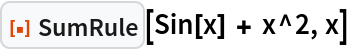Out=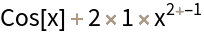### Scope (1)

If there is no explicit sum in the first argument of SumRule, one of the terms is taken to be 0:

 In:=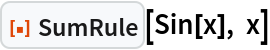Out=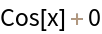### Options (3)

#### InactiveInnerDerivatives (1)

Setting "InactiveInnerDerivatives" to True inactivates the derivatives of the factors within the structure of the sum rule:

 In:=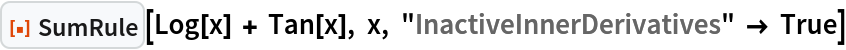Out=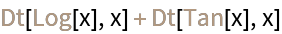#### PrintNapkin (1)

Setting "PrintNapkin" to True prints a grid with the side "napkin" work needed to apply the sum rule (i.e., identifying and computing the derivatives of each term):

 In:=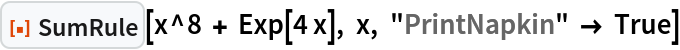Out=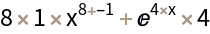#### PrintRule (1)

Setting "PrintRule" to True prints a general statement of the sum rule for derivatives along with general statements of the derivative rule needed to compute the derivative of each term:

 In:=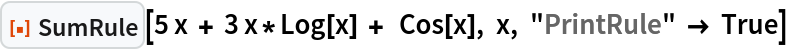Out=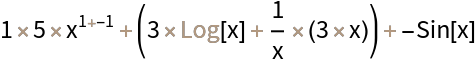## Publisher

Wolfram|Alpha Math Team

## Requirements

Wolfram Language 13.0 (December 2021) or above

## Version History

• 1.0.0 – 06 June 2023

## Author Notes

To view the full source code for SumRule, evaluate the following: